# Superposition Theorem

## Network Analysis Techniques

• #### Question 1

 Don’t just sit there! Build something!!

Learning to mathematically analyze circuits requires much study and practice. Typically, students practice by working through lots of sample problems and checking their answers against those provided by the textbook or the instructor. While this is good, there is a much better way.

You will learn much more by actually building and analyzing real circuits, letting your test equipment provide the “answers” instead of a book or another person. For successful circuit-building exercises, follow these steps:

1. Carefully measure and record all component values prior to circuit construction.
2. Draw the schematic diagram for the circuit to be analyzed.
3. Carefully build this circuit on a breadboard or other convenient medium.
4. Check the accuracy of the circuit’s construction, following each wire to each connection point, and verifying these elements one-by-one on the diagram.
5. Mathematically analyze the circuit, solving for all values of voltage, current, etc.
6. Carefully measure those quantities, to verify the accuracy of your analysis.
7. If there are any substantial errors (greater than a few percent), carefully check your circuit’s construction against the diagram, then carefully re-calculate the values and re-measure.

Avoid very high and very low resistor values, to avoid measurement errors caused by meter “loading”. I recommend resistors between 1 kΩ and 100 kΩ, unless, of course, the purpose of the circuit is to illustrate the effects of meter loading!

One way you can save time and reduce the possibility of error is to begin with a very simple circuit and incrementally add components to increase its complexity after each analysis, rather than building a whole new circuit for each practice problem. Another time-saving technique is to re-use the same components in a variety of different circuit configurations. This way, you won’t have to measure any component’s value more than once.

• #### Question 2

Suppose we have a single resistor powered by two series-connected voltage sources. Each of the voltage sources is “ideal,” possessing no internal resistance:Calculate the resistor’s voltage drop and current in this circuit.

Now, suppose we were to remove one voltage source from the circuit, replacing it with its internal resistance (0 Ω). Re-calculate the resistor’s voltage drop and current in the resulting circuit:Now, suppose we were to remove the other voltage source from the circuit, replacing it with its internal resistance (0 Ω). Re-calculate the resistor’s voltage drop and current in the resulting circuit:One last exercise: “superimpose” (add) the resistor voltages and superimpose (add) the resistor currents in the last two circuit examples, and compare these voltage and current figures with the calculated values of the original circuit. What do you notice?

• #### Question 3

Suppose we have a single resistor powered by two parallel-connected current sources. Each of the current sources is “ideal,” possessing infinite internal resistance:Calculate the resistor’s voltage drop and current in this circuit.

Now, suppose we were to remove one current source from the circuit, replacing it with its internal resistance (∞ Ω). Re-calculate the resistor’s voltage drop and current in the resulting circuit:Now, suppose we were to remove the other current source from the circuit, replacing it with its internal resistance (∞ Ω). Re-calculate the resistor’s voltage drop and current in the resulting circuit:One last exercise: “superimpose” (add) the resistor voltages and superimpose (add) the resistor currents in the last two circuit examples, and compare these voltage and current figures with the calculated values of the original circuit. What do you notice?

• #### Question 4

The Superposition Theorem is a very important concept used to analyze both DC and AC circuits. Define this theorem in your own words, and also state the necessary conditions for it to be freely applied to a circuit.

• #### Question 5

Explain in your own words how to apply the Superposition Theorem to calculate the amount of current through the load resistor in this circuit: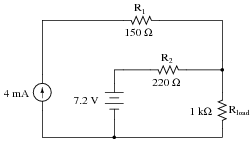• #### Question 6

The Superposition Theorem works nicely to calculate voltages and currents in resistor circuits. But can it be used to calculate power dissipations as well? Why or why not? Be specific with your answer.

• #### Question 7

Note that this circuit is impossible to reduce by regular series-parallel analysis:However, the Superposition Theorem makes it almost trivial to calculate all the voltage drops and currents: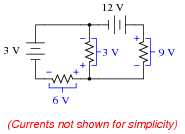Explain the procedure for applying the Superposition Theorem to this circuit.

• #### Question 8

Use the Superposition Theorem to calculate the amount of current going through the 55 Ω heater element. Ignore all wire and connection resistances, only considering the resistance of each fuse in addition to the heater element resistance: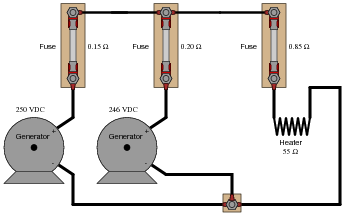• #### Question 9

Suppose a DC generator is powering an electric motor, which we model as a 100 Ω resistor:Calculate the amount of current this generator will supply to the motor and the voltage measured across the motor’s terminals, taking into account all the resistances shown (generator internal resistance rgen, wiring resistances Rwire, and the motor’s equivalent resistance).

Now suppose we connect an identical generator in parallel with the first, using connecting wire so short that we may safely discount its additional resistance:Use the Superposition Theorem to re-calculate the motor current and motor terminal voltage, commenting on how these figures compare with the first calculation (using only one generator).

• #### Question 10

Calculate the charging current through each battery, using the Superposition Theorem (ignore all wire and connection resistances - only consider the resistance of each fuse):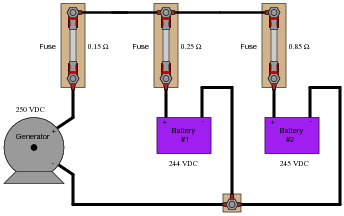• #### Question 11

Suppose we have a single resistor powered by two series-connected voltage sources. Each of the voltage sources is “ideal,” possessing no internal resistance:Calculate the resistor’s voltage drop and current in this circuit.

Now, suppose we were to remove one voltage source from the circuit, replacing it with its internal resistance (0 Ω). Re-calculate the resistor’s voltage drop and current in the resulting circuit:Now, suppose we were to remove the other voltage source from the circuit, replacing it with its internal resistance (0 Ω). Re-calculate the resistor’s voltage drop and current in the resulting circuit:One last exercise: “superimpose” (add) the resistor voltages and superimpose (add) the resistor currents in the last two circuit examples, and compare these voltage and current figures with the calculated values of the original circuit. What do you notice?

• #### Question 12

Suppose we have a single resistor powered by two parallel-connected current sources. Each of the current sources is “ideal,” possessing infinite internal resistance:Calculate the resistor’s voltage drop and current in this circuit.

Now, suppose we were to remove one current source from the circuit, replacing it with its internal resistance (∞ Ω). Re-calculate the resistor’s voltage drop and current in the resulting circuit:Now, suppose we were to remove the other current source from the circuit, replacing it with its internal resistance (∞ Ω). Re-calculate the resistor’s voltage drop and current in the resulting circuit:One last exercise: “superimpose” (add) the resistor voltages and superimpose (add) the resistor currents in the last two circuit examples, and compare these voltage and current figures with the calculated values of the original circuit. What do you notice?

• #### Question 13

A windmill-powered generator and a battery work together to supply DC power to a light bulb. Calculate the amount of current through each of these three components, given the values shown in the schematic diagram. Assume internal resistances of the generator and battery to be negligible:Ibatt = Ibulb = Igen =

• #### Question 14

A windmill-powered generator and a battery work together to supply DC power to a light bulb. Calculate the amount of current through each of these three components, given the values shown in the schematic diagram. Assume internal resistances of the generator and battery to be negligible:Ibatt = Ibulb = Igen =

• #### Question 15

Electrical signals are frequently used in industrial control applications to communicate information from one device to another. An example of this is motor speed control, where a computer outputs a speed command signal to a motor “drive” circuit, which then provides metered power to an electric motor: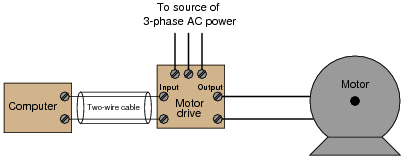Two common standards for analog control signals are 1-5 volts DC and 4-20 mA DC. In either case, the motor will spin faster when this signal from the computer grows in magnitude (1 volt = motor stopped, 5 volts = motor runs at full speed; or 4 mA = motor stopped, 20 mA = motor runs at full speed).

At first, it would seem as though the choice between 1-5 volts and 4-20 mA as control signal standards is arbitrary. However, one of these standards exhibits much greater immunity to induced noise along the two-wire cable than the other. Shown here are two equivalent schematics for these signal standards, complete with an AC voltage source in series to represent the “noise” voltage picked up along the cable’s length: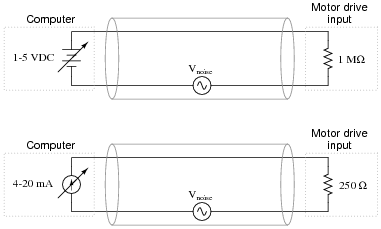Use the superposition theorem to qualitatively determine which signal standard drops the greatest amount of noise voltage across the motor drive input’s resistance, thereby most affecting the motor speed control.

• #### Question 16

Sketch the approximate waveform of this circuit’s output signal (Vout) on the screen of the oscilloscope: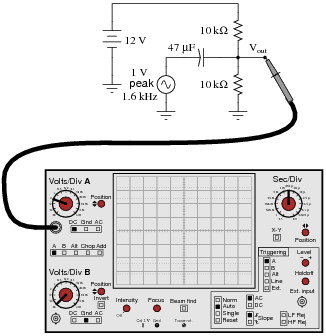Hint: use the Superposition Theorem!

• #### Question 17

Sketch the approximate waveform of this circuit’s output signal (Vout) on the screen of the oscilloscope: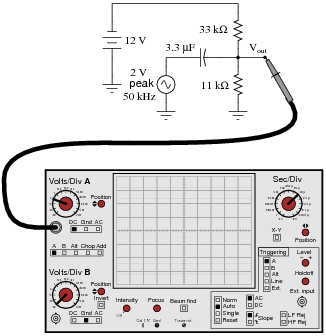Hint: use the Superposition Theorem!

### Related Content

Published under the terms and conditions of the Creative Commons Attribution License• K
KC Anoruo July 28, 2020

As a student, it will be better if these worksheets had not only answers but explanations as well so one can see where they went wrong.
Thank you.

Like.
•ituo October 28, 2020
i really don't know how 44v 0.15Ω could produce like 3.992 A so small current may be i really should leard SPICE or give up not like you have teacher to ask
Like.
•RK37 October 29, 2020
If you're having difficulty understanding one of these exercises, you could try posting a question in the AAC forum: https://www.allaboutcircuits.com/forums/
Like.